Courses

# Extra Questions and Practice Exercise- Introduction to Graphs Class 8 Notes | EduRev

## Mathematics (Maths) Class 8

Created by: Full Circle

## Class 8 : Extra Questions and Practice Exercise- Introduction to Graphs Class 8 Notes | EduRev

The document Extra Questions and Practice Exercise- Introduction to Graphs Class 8 Notes | EduRev is a part of the Class 8 Course Mathematics (Maths) Class 8.
All you need of Class 8 at this link: Class 8

Extra Questions
Question 1: Draw the points (5, 4) and (4, 5). Do they represent the same point?
Solution: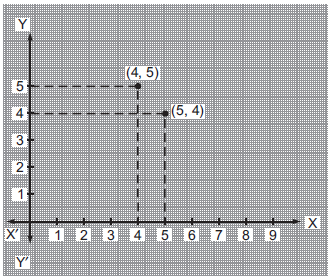From the graph, we observe that the points (5, 4) and (4, 5) are different, i.e. they represent different points.

Question 2: Draw a line passing through (2, 1) and (1, 2). Find the coordinates of the points at which this line meets the x-axis and y-axis.
Solution: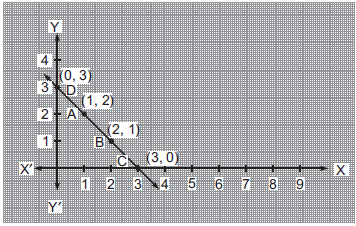On plotting the given points A(1, 2) and B(2, 1) on the same graph and joining them, we get a straight line AB. When we extend the line AB on both sides, we find that it intersects the x-axis at C(3, 0) and the y-axis at D(0, 3).

Question 3: Draw the graph for the following table of values of time (in hours) and distances (in km) covered by a car.

 Time (in hours) 7:00 8:00 9:00 10:00 Distance (in km) 60 120 180 240

From the graph, find:
(i) The distance covered by the car during the period 7:00 to 8:00.
(ii) At what time the car would have covered 180 km?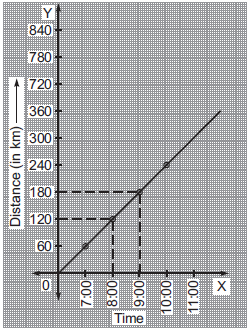Solution: By plotting the points (7:00, 60), (8:00, 120), (9:00, 180) and (10:00, 240), we get a straight line.
(i) Distance covered during 7:00 and 8:00
= (180 – 120) km
= 60 km
(ii) From the graph the car would have covered 180 km at 9:00.

Question 4: Find the coordinates of the vertices of DABC given in graph. Draw a triangle by taking vertices as A(5, 2), B(1, 2) and C(1, 5).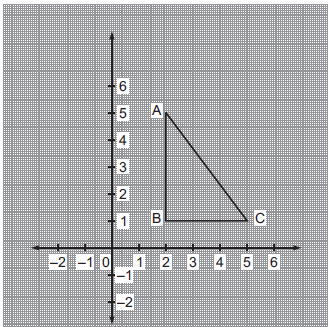Solution: From the graph, the vertices of DABC are: A, B and C. We find that
The co-ordinates of A are (2, 5).
The co-ordinates of B are (2, 1).
The co-ordinates of C are (5, 1).
The required triangle with vertices as A(5, 2), B(1, 2) and C(1, 5) is given in the following graph.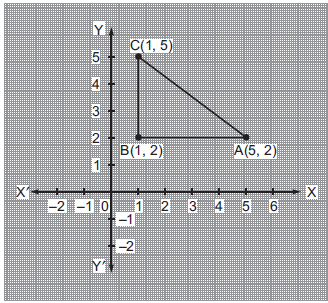Question 5: Following graph describes the movement of a car from a town A to town D. Study the graph and answer the following questions: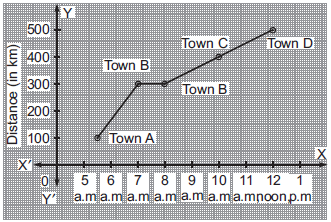(i) What is the distance between town A and town D?
(ii) What did the car start from town A?
(iii) Where did the car stop and for what duration?
(iv) How long did it take to go from town C to town D?
Solution: (i) The distance between the town A and town D is 500 km – 100 km = 400 km.
(ii) The car started from the town A at 5 a.m.
(iii) The car stopped at town B for 1 hour (7 a.m. to 8 a.m.).
(iv) The car reached at town D at 12 noon.
(v) The car look 2 hours to reach from town C to town D.

Question 6: Read the following ‘time-temperature’ graph of a place and answer the questions given below.
(i) What was the temperature at 7 a.m.?
(ii) When the temperature was maximum?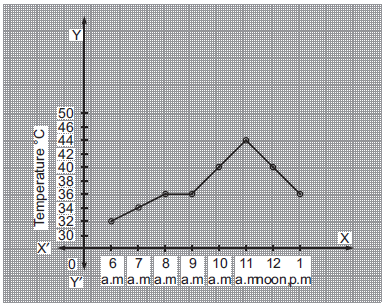(iii) When was the temperature 40°C?
(iv) During which period, the temperature remained constant?
Solution: (i) At 7 a.m., the temperature was 34° C.
(ii) The maximum temperature (44° C) was at 11 a.m.
(iii) At 10 a.m., the temperature was 40° C.
(iv) The temperature remained constant during 8 a.m. to 9 a.m.

Practice Exercise
Question 1. The graph shows the temperature of a patient recorded before noon. Read it and answer the following questions.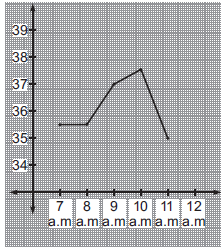(i) What was patient’s temperature at 9 a.m.?
(ii) What the highest temperature of the patient?
(iii) When was the patient’s temperature lowest?
(iv) During which period, the patient’s temperature remained constant?
(ii) 37.5° C
(iii) 11 a.m.
(iv) 7 a.m. to 8 a.m.

Question 2. The graph shows the yearly sales figure of a shoe manufacturing company.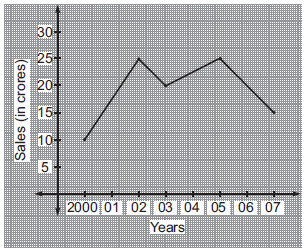(i) What were the sales in 2000?
(ii) In which year the sales were maximum?
(iii) What is the difference between the sales in the year 2003 and 2005?
(ii) in 2002 and 2005
(iii) 0

Question 3. Plot the points A(4, 3), B(4, 0), (4, –2), (4, 6) and join them. Do they lie on the same line?

Question 4. Draw a line passing through (4, 5) and (5, 4). Find the coordinates of the points at which this line meets the x-axis and y-axis.
Answer: x-axis: (9, 0) and y-axis: (0, 9)

Question 5. Look at the graph of a rectangle in the figure. What are the coordinates of its vertices?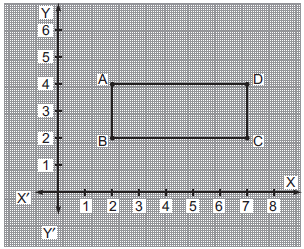Answer:  A(2, 4), B(2, 2), C(7, 2), D(7, 4)

Question 6. Draw a ‘deposit-interest’ graph for the following data:

 Deposit (in Rs) 5000 6000 7000 8000 9000 Simple interest(in Rs) for 1 year 400 480 560 640 720

From the graph, find the interest on Rs 7500 for 1 year.

,

,

,

,

,

,

,

,

,

,

,

,

,

,

,

,

,

,

,

,

,

;